# Compound Wound DC Motor or DC Compound Motor

A compound wound DC motor (also known as a DC compound motor) is a type of self-excited motor, and is made up of both series the field coils S1 S2 and shunt field coils F1 F2 connected to the armature winding as shown in the figure below.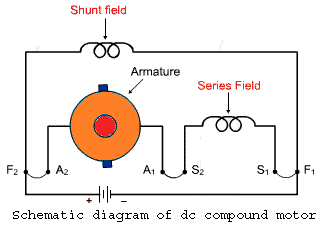Both the field coils provide for the required amount of magnetic flux, that links with the armature coil and brings about the torque necessary to facilitate rotation at the desired speed. As we can understand, a compound wound DC motor is basically formed by the amalgamation of a shunt wound DC motor and series wound DC motor to achieve the better off properties of both these types. Like a shunt wound DC motor is bestowed with an extremely efficient speed regulation characteristic, whereas the DC series motor has high starting torque.

So the compound wound DC motor reaches a compromise in terms of both these features and has a good combination of proper speed regulation and high starting torque.

Though its starting torque is not as high as in case of DC motor, nor is its speed regulation as good as a shunt DC motor. Overall characteristics of DC shunt motor falls somewhere in between these 2 extreme limits. You can learn more about motors by studying our electrical MCQs.

## Types of Compound Wound DC Motor

The compound wound DC motor can further be subdivided into 2 major types on the basis of its field winding connection with respect to the armature winding, and they are:

### Long Shunt Compound Wound DC Motor

In case of long shunt compound wound DC motor, the shunt field winding is connected in parallel across the series combination of both the armature and series field coil, as shown in the diagram below.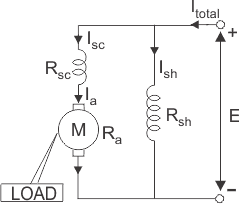### Voltage and Current Equation of Long Shunt Compound Wound DC Motor

Let E and Itotal be the total supply voltage and current supplied to the input terminals of the motor. And Ia, Ise , Ish be the values of current flowing through armature resistance Ra, series winding resistance Rse and shunt winding resistance Rsh respectively.
Now we know in shunt motor,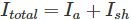And in series motor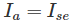Therefore, the current equation of a compound wound DC motor is given by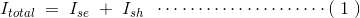And its voltage equation is,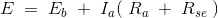### Short Shunt Compound Wound DC Motor

In case of short shunt compound wound DC motor, the shunt field winding is connected in parallel across the armature winding only. And series field coil is exposed to the entire supply current, before being split up into armature and shunt field current as shown in the diagram below.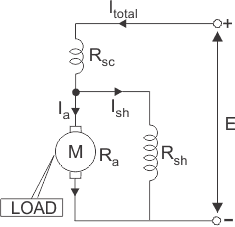### Voltage and Current Equation of Short Shunt Compound Wound DC Motor

Here also let, E and Itotal be the total supply voltage and current supplied to the input terminals of the motor. And Ia, Ise, Ish be the values of current flowing through armature resistance Ra, series winding resistance Rse and shunt winding resistance Rsh respectively.
But from the diagram above we can see,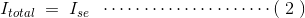Since the entire supply current flows through the series field winding.
And like in the case of a DC shunt motor,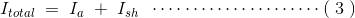Equation (2) and (3) gives the current equation of a short shunt compound wound DC motor.
Now for equating the voltage equation, we apply Kirchoff’s law to the circuit and get,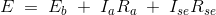But since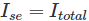Thus the final voltage equation can be written as,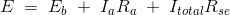Apart from the above mentioned classification, a compound wound DC motor can further be sub divided into 2 types depending upon excitation or the nature of compounding. i.e.

#### Cumulative Compounding of DC Motor

A compound wound DC motor is said to be cumulatively compounded when the shunt field flux produced by the shunt winding assists or enhances the effect of main field flux, produced by the series winding.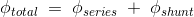#### Differential Compounding of DC Motor

Similarly a compound wound DC motor is said to be deferentially compounded when the flux due to the shunt field winding diminishes the effect of the main series winding. This particular trait is not really desirable, and hence does not find much of a practical application.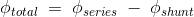The net flux produced in this case is lesser than the original flux and hence does not find much of a practical application.
The compounding characteristic of the self excited DC motor is shown in the figure below.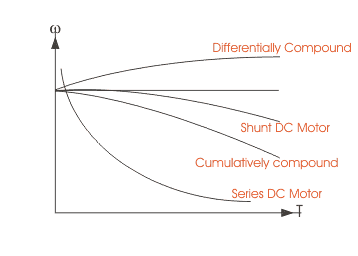Want To Learn Faster? 🎓
Get electrical articles delivered to your inbox every week.
No credit card required—it’s 100% free.